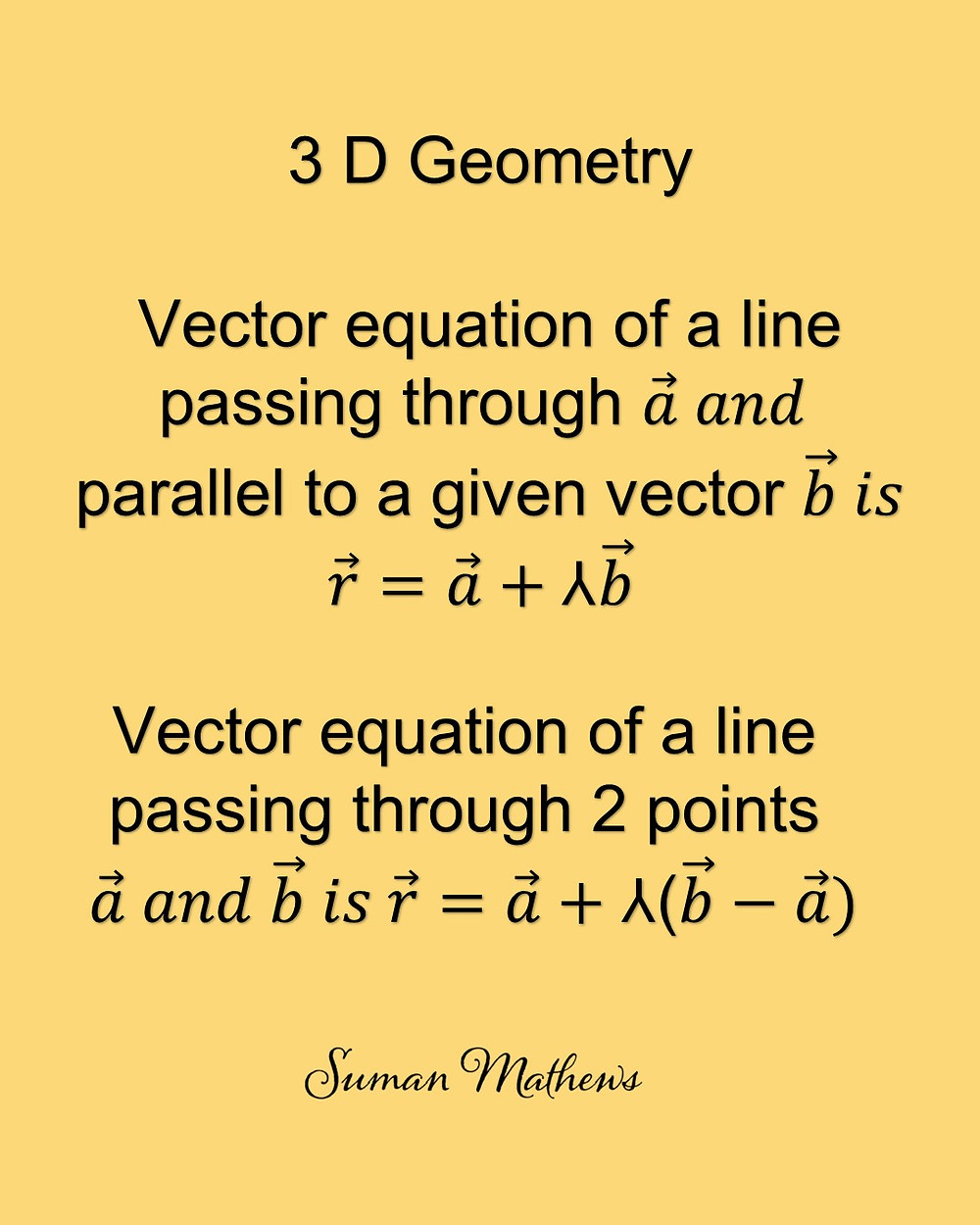Search

# Learn how to calculate the Vector equation of a line

Learn how to calculate the vector equation of a line passing through a point and parallel to a given vector and how to find the vector equation of a line passing through 2 points. Class 12 Mathematics students, please note, this is a part of 3 Dimensional Geometry. Do you know the 2 forms of a line? You could email me your queries at mathmadeeasy22@gmail.com